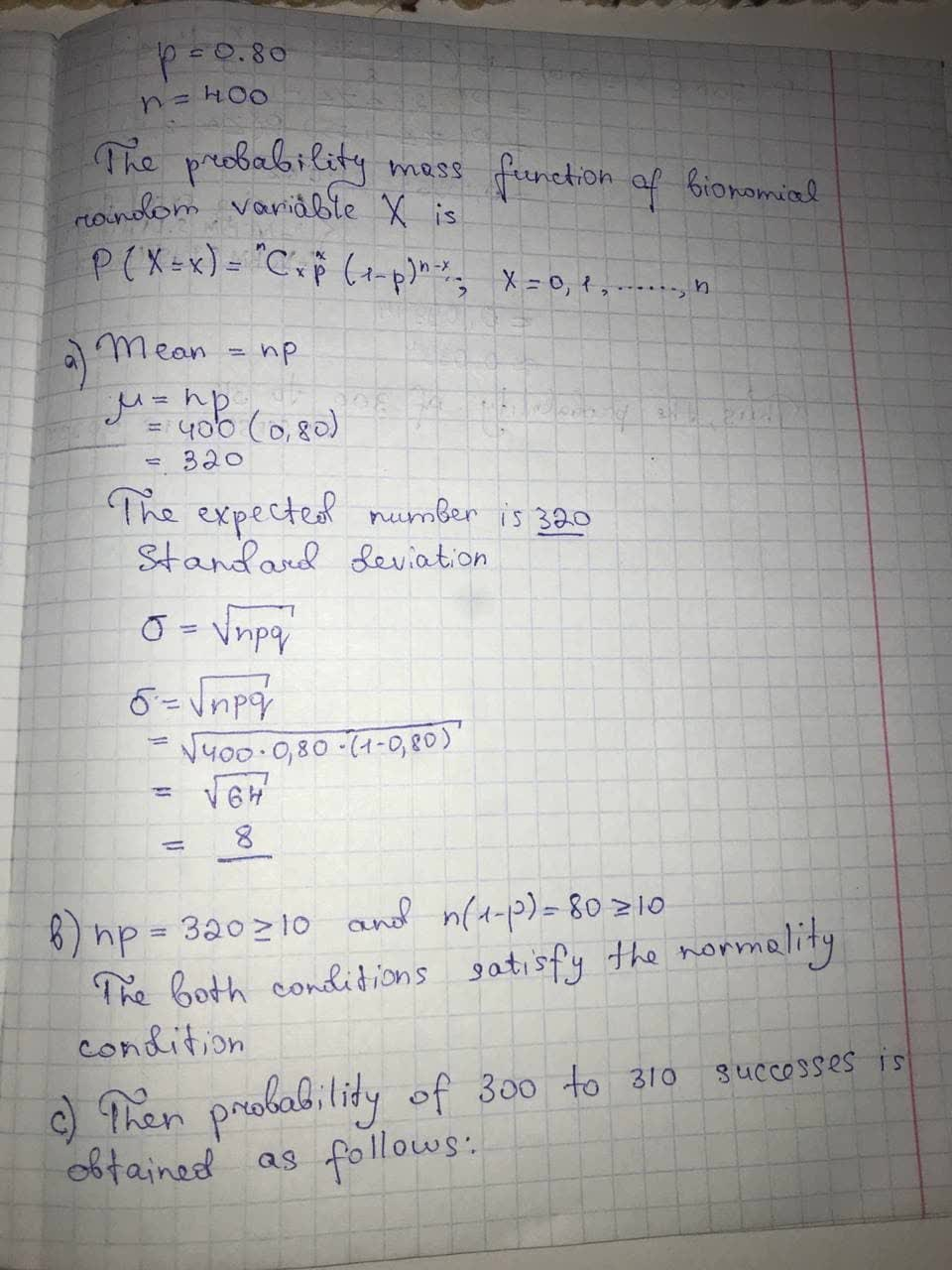Assume a binomial probability distribution has p = 0.80 and n = 400.a) what is the mean and standard deviationnaivlingr 2021-09-07 Answered
Assume a binomial probability distribution has p = 0.80 and n = 400.
a) what is the mean and standard deviation
b) is this situation one in which binomial probabilities can be approximated by the normal probability distribution? Explain.
c) what is the probability of 300 to 310 successes? Use the normal approximation of the binomial distribution to answer this question. (Round your answer to four decimal places.)

• Questions are typically answered in as fast as 30 minutes

Solve your problem for the price of one coffee

• Math expert for every subject
• Pay only if we can solve itAniqa O'Neill